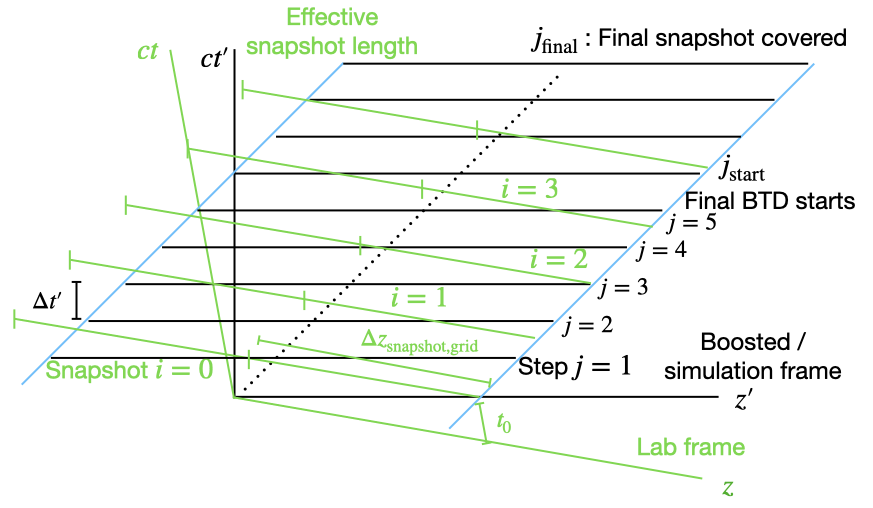# FAQ¶

This section lists frequently asked usage questions.

## What is MPI thread support level?¶

We report this in output on startup together with other information.

That is the MPI support for threaded execution, e.g., with OpenMP or system threads.

We currently only use this for optional, async IO with AMReX plotfiles. In the past, requesting MPI threading support had performance penalties, but we have not seen such anymore on recent systems. Thus, we request it by default but you can overwrite it with a compile time option if it ever becomes needed.

## How do I suppress tiny profiler output if I do not care to see it?¶

Via AMReX_TINY_PROFILE=OFF (see: build options and then AMReX build options). We change the default in cmake/dependencies/AMReX.cmake.

Note that the tiny profiler adds literally no overhead to the simulation runtime, thus we enable it by default.

## What design principles should I keep in mind when creating an input file?¶

Leave a cushion between lasers, particles, and the edge of computational domain. The laser antenna and plasma species zmin can be less than or greater than the geometry.prob_hi, but not exactly equal.

## What do I need to know about using the boosted frame?¶

The input deck can be designed in the lab frame and little modification to the physical set-up is needed – most of the work is done internally. Here are a few practical items to assist in designing boosted frame simulations:

• Ions must be explicitly included

• Best practice is to separate counter-propagating objects; things moving to the right should start with $$z <= 0$$ and things stationary or moving to the left (moving to the left in the boosted frame) should start with $$z > 0$$

• Don’t forget the general design principles listed above

• The boosted frame simulation begins at boosted time $$t'=0$$

• Numerics and algorithms need to be adjusted, as there are numerical instabilities that arise in the boosted frame. For example, setting particles.use_fdtd_nci_corr=1 for an FDTD simulation or setting psatd.use_default_v_galilean=1 for a PSATD simulation. Be careful as this is overly simplistic and these options will not work in all cases. Please see the input parameters documentation and the examples for more information

An in-depth discussion of the boosted frame is provided in the moving window and optimal Lorentz boosted frame section.

## What about Back-transformed diagnostics (BTD)?¶Fig. 5 [fig:BTD_features] Minkowski diagram indicating several features of the back-transformed diagnostic (BTD). The diagram explains why the first BTD begins to fill at boosted time $$t'=0$$ but this doesn’t necessarily correspond to lab time $$t=0$$, how the BTD grid-spacing is determined by the boosted time step $$\Delta t'$$, hence why the snapshot length don’t correspond to the grid spacing and length in the input script, and how the BTD snapshots complete when the effective snapshot length is covered in the boosted frame.

Several BTD quantities differ slightly from the lab frame domain described in the input deck. In the following discussion, we will use a subscript input (e.g. $$\Delta z_{\rm input}$$) to denote properties of the lab frame domain.

• The first back-transformed diagnostic (BTD) snapshot may not occur at $$t=0$$. Rather, it occurs at $$t_0=\frac{z_{max}}c \beta(1+\beta)\gamma^2$$. This is the first time when the boosted frame can complete the snapshot.

• The grid spacing of the BTD snapshot is different from the grid spacing indicated in the input script. It is given by $$\Delta z_{\rm grid,snapshot}=\frac{c\Delta t_{\rm boost}}{\gamma\beta}$$. For a CFL-limited time step, $$\Delta z_{\rm grid,snapshot}\approx \frac{1+\beta}{\beta} \Delta z_{\rm input}\approx 2 \Delta z_{\rm input}$$. Hence in many common use cases at large boost, it is expected that the BTD snapshot has a grid spacing twice what is expressed in the input script.

• The effective length of the BTD snapshot may be longer than anticipated from the input script because the grid spacing is different. Additionally, the number of grid points in the BTD snapshot is a multiple of <BTD>.buffer_size whereas the number of grid cells specified in the input deck may not be.

• The code may require longer than anticipated to complete a BTD snapshot. The code starts filling the $$i^{th}$$ snapshot around step $$j_{\rm BTD start}={\rm ceil}\left( i\gamma(1-\beta)\frac{\Delta t_{\rm snapshot}}{\Delta t_{\rm boost}}\right)$$. The code then saves information for one BTD cell every time step in the boosted frame simulation. The $$i^{th}$$ snapshot is completed and saved $$n_{z,{\rm snapshot}}=n_{\rm buffers}\cdot ({\rm buffer\ size})$$ time steps after it begins, which is when the effective snapshot length is covered by the simulation.

## What kinds of RZ output do you support?¶

In RZ, supported detail of RZ output depends on the output format that is configured in the inputs file.

openPMD supports output of the detailed RZ modes and reconstructs representations on-the-fly in post-processing, e.g, in openPMD-viewer or other tools. For some tools, this is in-development.

AMReX plotfiles and other in situ methods output a 2D reconstructed Cartesian slice at $$\theta=0$$ by default (and can opt-in to dump raw modes).